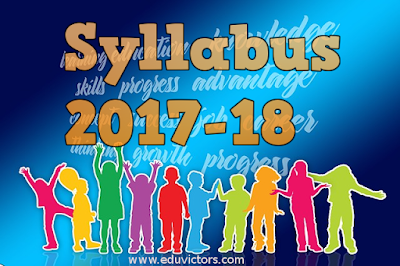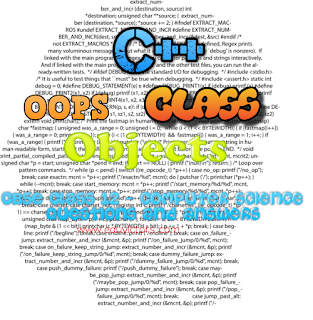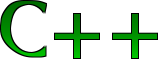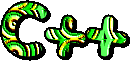Pages

Showing posts with label class12-computerScience. Show all posts
Showing posts with label class12-computerScience. Show all posts

C++ PracticalClass Design Snippet-2

Question 2: Write a declaration for a class Person which has the following:
• data members name, phone
• set and get functions for every data member
• a display function
• a destructor

(i) For the Person class above, write each of the constructors, the assignment operator and the getName member functions. Use member initialization list as often as possible

(ii) Given the Person class above, write the declaration for the class Spouse that inherits from Person and does the following:
• has an extra data member spouseName
• redefines the display member function

(iii) For the Spouse class above, write each of the constructors and display member, functions. Use member initialization lists as often as possible.C++ PracticalClass Design Snippet-1

Question 1: Imagine a publishing company that markets both book and audio-cassette versions of its works. Create a class publication that stores the title (a string) and price (type float) of a publication. From this class derive two classes: book, which adds a page count (type int) and tape, which adds a playing time in minutes (type float). Each of these three classes should have a getdata() function to get its data from the user and a putdata() function to display its data. Write a main() program to test the book and tape classes by creating instances of then displaying the data with putdata().Data Transmission Media

Computer Networking

Class 12 - Computer Science / Informatics Practices

Q & A

Q: Define transmission media.

Answer: The means or channel through which we send our data from one place to another are called transmission media.

Q: What are different categories of transmission media?

Guided or Wired Media- Examples are
Twisted Pair Cable
Co-axial cable
Fibre active cable

Unguided or Wireless Media - Examples are:
Satellite
Microwave
Wifi

Q: Define baud.

Answer: Baud is used as measurement for the information carry of a communication channel. It is measured in Kbps (kilobits Per Second), KBPS (Kilo Byte Per Second), and Mbps (Mega bits Per Second )Computer Networking

Q and A

Q1: What is a computer network?

Answer: A computer network is a collection of interconnected computers. Two computers are said to be interconnected if they are capable of sharing hardware, software and exchanging information.

Q2: Name the architectures that are involved in network design?

Peer to peer (P2P)
Client - Server
Hybrid of client-server and P2PComputer Science (C++ / Python) -Syllabus (2017-18)

CBSE Class 11 and 12 Computer Science

Here presents the prescribed syllabus for Class 11 and Class 12 Computer Science for the curriculum 2017-18.C++ - Constructors and Destructors

CBSE Class 12 - Computer Science - Questions and Answers

Q1: What is a constructor? How it is different from other member functions?

Answer: A constructor is a special member function that must be defined with the same name as the class and it is used to initialize the data members of the class. Constructor is invoked automatically when an object of a class is created. Constructors unlike other functions cannot return values, so they cannot specify a return type (not even void ).Generally, constructors are declared public.

Q2: Name the types of constructors.

Default Constructor (No argument constructor)
Copy ConstructorC++ - OOPS, Class and Objects

CBSE Class 12 - Computer Science - Questions and Answers

Q1: Define class.

Answer: A class is collection of data (data member) and functions (member functions or methods)
working on the data. It can be seen as a blueprint for the object. Data define the attributes and methods define behaviours of the class.

Q2: Does memory allocated when a class is created?

Answer: No memory is allocated when a class is created. Memory is allocated only when an object is created.

Q3: Which keyword is used to begin the definition of a class?Saturday, 14 January 2017

CBSE Class 12 - Computer Science- Marking Scheme of Sample Question Paper (2016-17) (#cbseclass12papers)

CBSE Class 12 - Computer Science- Marking Scheme of Sample Question Paper (2016-17)Saturday, 7 January 2017

CBSE Class 12 - Computer Science- Sample Question Paper (2016-17) (#CBSEClass12Papers)

CBSE Class 12 - Computer Science- Sample Question Paper (2016-17)Saturday, 23 January 2016

CBSE Class 12 - Computer Science- Marking Scheme- Sample Question Paper (2015-16)

CBSE Class 12 - Computer Science- Marking Scheme- Sample Question Paper (2015-16)Sunday, 14 June 2015

There is 1 or 2 Marks questions based on function mapping to header filesQ1(CBSE 2012): Write the names of the header file to which of the following belong:

(i) sqrt( )
(ii) randomize()

(ii) stdlib.h

Q2: Name the header file that will be needed for the following code:

```   void main()
{
char word [] = "Board Examination";
cout << setw(20) << word;
}
```

Answer: iostream.h (for cout), iomanip.h (for setw)

Q3(CBSE 2012): Which C++ header file(s) are especially required to be included to run the following C++ code. (Note: Do not include any header which is/are not required):

```   void main()
{
char TEXT[] = "something";
cout << "Remaining SMS chars: " << 160 - strlen(TEXT) << endl;
}
```

Answer: iostream.h (for cout), string.h (for strlen)

Q4(CBSE 2013): Observe the following C++ code and write name(s) of the header file(s) which will be essentially required to run in a C++ compiler:

```    void main()
{
int number;
cin >> number;
if (abs(number) == number)
cout << "positive" << endl;
}
```

Answer: iostream.h (for cin, cout), math.h (for abs)C++ Inheritance Questions  Asked in Board Examination

Q&AQ1(CBSE 2004): What is inheritance? Give an example in C++ to show its implementation in C++.

Answer: Inheritance is the process of forming a new class from an existing or base class. The base class is also known as parent class or super class. It allows the new class (called derived class) to inherit properties of its parent class.Inheriatnce helps in reusability of code.
For example, a class Truck inherits from class Automobiles which itself inherits from another class vehicles.

Q2(CBSE 2004): Given the following class definitions, answer the following questions.

```   class Book
{
char title;
char author;
int no_of_pages;
public:
void show( );
};

class TextBook : private Book
{
int no_of_chapters, no_of_assignments;
protected:
int standard;
public:
void showtextbook( );
};

class PhysicsBook : public TextBook
{
char topic;
public:
void showphysicsbook( );
};
```

(i) Name the members, which can be accessed from the member functions of class PhysicsBook.
(ii) Name the members, which can be accessed by an object of class TextBook.
(iii) Name the members which can be accessed by an object of class Physicsbook.
(iv) What will be the size of an object (in bytes) of class Physicsbook?

(iv)20 + 20 + 2 + 2 + 2 + 2 + 20 = 68 bytes.

Q3 (CBSE 2013): Consider the following c++ code and answer the questions (i) to (iv):Computer Science (C++) - Class Design

Questions Asked in Board Papers on Class DesignQ1(CBSE 2013):  Define a class Tourist in C++ with the following specifications:
Data Members:
• CarNo - to store bus number
• Origin - to store Place name
• Destination - to store Place name
• Type - to store Car Type such as 'E' for Economy
• Distance - to store the Distance in Kilometers
• Charge - to store the Car Fare
Member Functions:
• A constructor function to initialize Type as 'E' and Freight as 250
• A function CalcCharge( ) to calculate Fare as per the following criteria:
 Type Charge 'E' 16*Distance 'A' 22*Distance 'L' 30*Distance

• A function Enter( ) to allow user to enter values for CarNo, Origin, Destination, Type and Distance. Also this function should call CalcCharge( ) to calculate Fare.
• A function Show( ) to display the content of all the data members on screen.
``` const int BUFFSIZE = 40;
class Tourist
{
// private data members
int carNum;
char origin[BUFFSIZE];
char destination[BUFFSIZE];
char carType;
int distance;
int charges;
public:
// member functions
// constructor
Tourist()
{
carType = 'E';
charges = 250;
}

// calculate charges
void CalcCharges()
{
if (Type == 'E')
{
charges = 16*distance;
}
else if (Type == 'A')
{
charges = 22*distance;
}
else if (Type == 'C')
{
charges = 30*distance;
}
};

// enter values
void Tourist::Enter()
{
cout << "Enter CarNo, Origin, Destination, Type, Distance:";
cin >> carNum;
gets(origin);
gets(destination);
cin >> carType >> Distance;
CalcCharges();
}

// show/display details
void Show()
{
cout << "Car No: " << carNum
<< "\nOrigin: " << origing
<< "\nDestination: " << destination
<< "\nType: " << carType
<< "\nDistance: " << distance
<< "\nCharges: " << charges << endl;
}
};```

Q2(CBSE 2012): Define a class Flat in C++ with the following description:
Private Members:Class 12Computer ScienceSample Q Paper (2014-15)

 CLASS-XII COMPUTER SCIENCE (Subject Code 083) SAMPLE PAPER 2014 - 15 Time allowed : 3 hours Maximum Marks: 70 Instructions: (i) All questions are compulsory. (ii) Programming Language: Section A C+ +. (iii) Programming Language : Section B Python. (iv) Answer either Section A or B, and Section C is compulsory. Section A (C++) Q1 (a) Differentiate between ordinary function and member functions in C++. Explain with an example.  (b) Write the related library function name based upon the given information in C++. (i) Get single character using keyboard. This function is available in stdio.h file. (ii) To check whether given character is alpha numeric character or not. This function is available in ctype.h file.  (c) Rewrite the following C++ program after removing all the syntactical errors (if any), underlining each correction. : ```include #define PI=3.14 void main( ) { float r;a; cout<<’enter any radius’; cin>>r; a=PI*pow(r,2); cout<< ”Area=” << a }```  (d) Write the output from the following C++ program code: ```#include #include ``` ```void strcon(char s[]) { for(int i=0,l=0;s[i]!='\0';i++,l++); for(int j=0; j